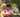### Max Counters

January 23, 2020

Max Counters

You are given N counters, initially set to 0, and you have two possible operations on them:
increase(X) − counter X is increased by 1,
max counter − all counters are set to the maximum value of any counter.

A non-empty array A of M integers is given. This array represents consecutive operations: • if A[K] = X, such that 1 ≤ X ≤ N, then operation K is increase(X),
• if A[K] = N + 1 then operation K is max counter.

For example, given integer N = 5 and array A such that: (5, [3, 4, 4, 6, 1, 4, 4] )
A = 3
A = 4
A = 4
A = 6
A = 1
A = 4
A = 4
the values of the counters after each consecutive operation will be:
(0, 0, 1, 0, 0)
(0, 0, 1, 1, 0)
(0, 0, 1, 2, 0)
(2, 2, 2, 2, 2)
(3, 2, 2, 2, 2)
(3, 2, 2, 3, 2)
(3, 2, 2, 4, 2)
The goal is to calculate the value of every counter after all operations.

Write a function: function solution(N, A);
that, given an integer N and a non-empty array A consisting of M integers, returns a sequence of integers representing the values of the counters. Result array should be returned as an array of integers.

For example, given: [3, 4, 4, 6, 1, 4, 4]
A = 3
A = 4
A = 4
A = 6
A = 1
A = 4
A = 4
the function should return [3, 2, 2, 4, 2], as explained above.

Write an efficient algorithm for the following assumptions:
• N and M are integers within the range [1..100,000];
• each element of array A is an integer within the range [1..N + 1].

``````function solution(N, A) {
// write your code in JavaScript (Node.js 8.9.4)
const counters = []
let i = 0
let j = 0
let max = 0
let update = 0
for(j=0; j<N; j++) counters[j] = 0  // initialized with zeros
for(i=0; i<A.length; i++){
if(A[i]===N+1){
max = update
//max = Math.max(...counters) bad performance for large array
//for(j=0; j<N; j++) counters[j] = max
}
//if(A[i]>=1 && A[i]<=N) counters[ A[i]-1 ] ++
if(A[i]>=1 && A[i]<=N){
if(counters[ A[i]-1 ]>max) counters[ A[i]-1 ] ++
else counters[ A[i]-1 ] = max + 1
if(counters[ A[i]-1 ]>update) update = counters[ A[i]-1 ]
}

//console.log(counters)
}

for(j=0; j<N; j++){
if(counters[j]<max) counters[j]=max
}

return counters
}``````Posted by Tai Lu ( 呂台生 ) who's building useful things.You should follow him on Twitter